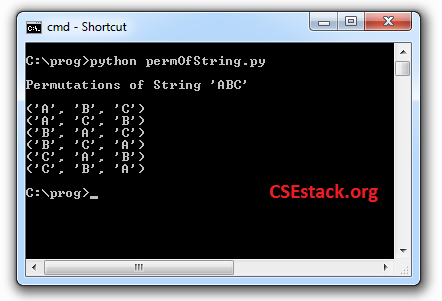# How to Get all the Permutations of String in Python using itertools?

Till now I have shared many Python programming examples. I am little surprised as I missed to write about permutations even though it is very popular.

For instance, you have to accomplish 5 jobs. You can execute these jobs in any order as you find productive. To find the best order, you have to get all the possible orders of Job. By comparing all those possible orders you can choose the best one. So the ordering of the jobs here is nothing but the permutation.

Let’s see…

### What is Permutation?

The permutation is nothing but the rearrangement of the elements in the set out of all possible orders.

Let’s take a string. It is a set of characters (letters).

If you have string ‘ABCD’; ‘BACD’, ‘ABDC’… are the permutations.

The number of permutation for the given set= n! (factorial of n)

– Where n is a number of elements in the given set.

In the above example, the length of the string ‘ABCD’ is 4. The number of permutation possible with this string is 4! = 24.

## Getting all the Permutations of String in Python Program:

For permutations, we can use backtracking technique. But if you are using Python, we have an inbuilt module to generate all valid permutations for the given object.

In this article, I will share a simple line of code to generate all the permutations of the string.

It’s not difficult as Python is ever mean to be the easiest language to learn.

You can use an existing Python module named itertools. This module comes with function permutations(). With this function, it is pretty easy to get all the permutations of string in Python.

```import itertools
print "\nPermutations of String 'ABC'\n"
for p in itertools.permutations('ABC'):
print(p)
```

This code will give full-length permutations for the elements. To print all the permutations, you just need to loop over it.

The output of a program:All the output permutations will be in lexicographic sort order.

If you look at the output you can see the last permutation is nothing but the reverse of the input string.

So, Reversing a string in Python returns output string which is one of the permutations of the given string.

If you want to get specific length permutation, you can pass the number of char in permutations function.

Here is code. It will print all the permutations for length 2.

```import itertools
for p in itertools.permutations('ABCD', 2):
print(p)
```

### Getting Permutation as Python List:

As we know the list is widely used and most prominent data structure in Python, you can convert all the permutation to list.

```from itertools import permutations
listPerm = list(permutations(range(1, 4)))
print listPerm
```

Here we are using range() function to choose the set of elements to find the permutations.

Now you can try permutations of string in Python to explore further or to make some awesome thing.

• Take the input from the user as a string and reply back with all possible permutation.
• You can create a small game. Ask a user to predict any permutation for the given set of elements. The user possibility of chosen right permutation is 1/(n!).

Use the comment section if you want to discuss anything related to this topic.

1.Mandar Kiran says:

itertools is a very good module to use. Earlier I was doing it with a manual code.

1.Aniruddha Chaudhari says:

Indeed!

2.Shalini says:

Thanks for writing this code; I was looking for this code to use in our project. It is very easy.

1.Aniruddha Chaudhari says:

You’re welcome!

3.Oyelayo says:

Keep on the good work. You are making me to love python more, even though I am a beginner

1.Aniruddha Chaudhari says:

Thanks mate! It motivates me.